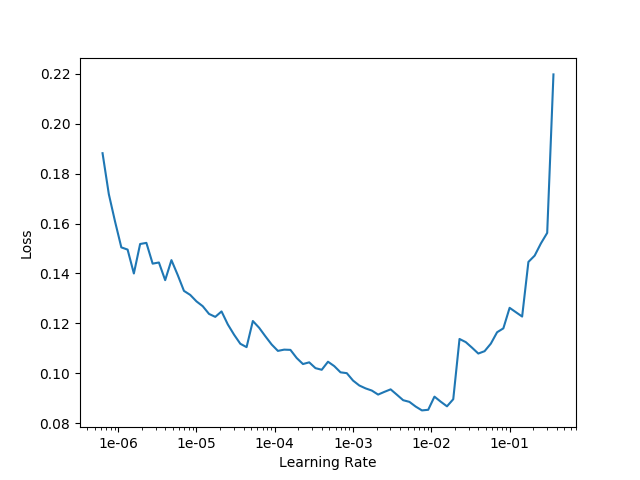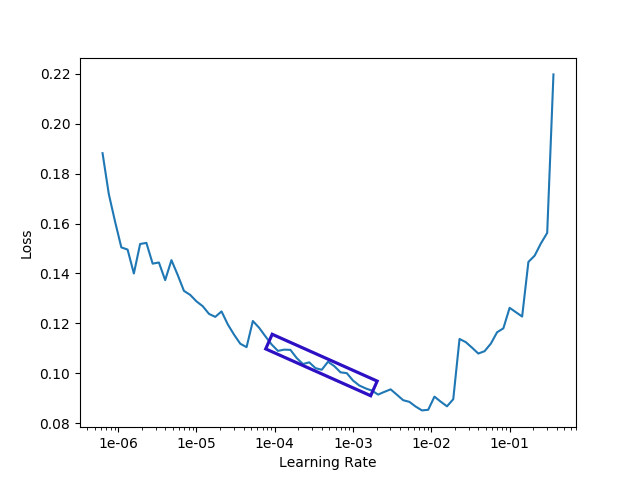# How to choose the learning_rate

If my first training results in a learning rate curve like this, how should I choose the learning rate range for the second training：I would generally go with somewhere in the box I’ve added below:You could go above or below that depending on whether this is the start of training or later, etc.

It’s still more a subjective science rather than math though at this point but hope that gives you some ideas.

1 Like

Thank you for your answer. Could you tell me how you chose this learning rate

Do you choose the part where the loss is smaller and the drop is faster and smoother

Based off of my experience, it seems like the optimal learning rate is the ideal combination of:

1). Steep negative gradient (negative slope)
2). Minimal loss
3). Curve smoothness

Based on the image you posted above, try experimenting with both:

`learn.fit_one_cycle(4, max_lr=slice(1e-4,1e-3))`

and

`learn.fit_one_cycle(4, max_lr=slice(1e-3,1e-2))`

2 Likes

Sure - I basically looked at a combination of:
1 - lowest point and then back off 10x at least to start. (i.e. if low is 1e-2, then I would not even consider anything higher than 1e-3 (10x).

2 - Angle of the slope, where steeper is better but…also how long does the slope run for.

There are some caveats though - if you are only training the head, you can be more aggressive than if training the whole network.
In addition, if this is the very start of training then you can/should lean towards more aggressive rates vs. later in training I tend to be more conservative.

Hopefully in the near future SLS will be ready (Stochastic line search optimizer) and then you won’t need to worry about lr, but for now hope the above is helpful!
Less

2 Likes